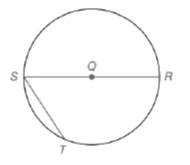Chapter 6.1, Problem 10EElementary Geometry For College St...

7th Edition
Alexander + 2 others
ISBN: 9781337614085

Solutions

Chapter
SectionElementary Geometry For College St...

7th Edition
Alexander + 2 others
ISBN: 9781337614085
Textbook Problem

Given: S T = 1 2 ( S R )  in  ⊙ Q   S R ¯  is a diameterFind: a) m S T ⌢ b) m T R ⌢ c) m S T R ⌢ d) m ∠ S (HINT: Dra Q T ¯ . )

To determine

a)

To calculate:

The mST from the provided figure.

Explanation

Given:

In a circle Q, ST=12(SR) where SR¯ is the diameter.

Theorem used:

The measure of an inscribed angle of a circle is one-half the measure of its intercepted arc.

An angle inscribed in a semicircle is a right angle.

Calculation:

From the figure we see that STR is a semicircle. Join TR¯ as shown below

We know that the angle inscribed in a semicircle is a right angle. Therefore STR=90.

Given that ST=12(SR).

In a right triangle STR, the hypotenuse is twice as longer than the shorter leg.

Hence the angles in right triangle STR are 30,60,90

To determine

b)

To calculate:

The mTR from the provided figure.

To determine

c)

To calculate:

The mSTR from the provided figure.

To determine

d)

To calculate:

The mS from the provided figure.

Still sussing out bartleby?

Check out a sample textbook solution.

See a sample solution

The Solution to Your Study Problems

Bartleby provides explanations to thousands of textbook problems written by our experts, many with advanced degrees!

Get Started

In Exercises 2124, factor each expression completely. 23. 16 x2

Applied Calculus for the Managerial, Life, and Social Sciences: A Brief Approach

Differentiate the function. y=x+xx2

Single Variable Calculus: Early Transcendentals, Volume I

Evaluate the expression sin Exercises 116. (23)2

Finite Mathematics and Applied Calculus (MindTap Course List)

True or False: n=1(1)nnn+3 converges.

Study Guide for Stewart's Single Variable Calculus: Early Transcendentals, 8th